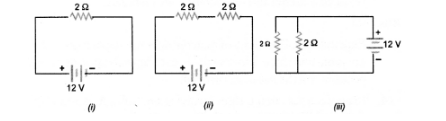# In the following circuits shown in figure,

Question:

In the following circuits shown in figure, power produced in the resistor or combination of resistors connected to a 12V battery will be(a) same in all cases

(b) minimum in case (i)

(c) maximum in case (ii)

(d) maximum in case (iii)

Solution:

(d).

Explanation: Power produced in case $(i)$

$=\frac{\mathrm{V}^{2}}{\mathrm{R}}=\frac{144}{2}=77 \mathrm{~J} \mathrm{~s}^{-1}$

Power produced in case $(i i)=\frac{\mathrm{V}^{2}}{\mathrm{R}}=\frac{144}{4}=36 \mathrm{Js}^{-1}$

Power produced in case (iii) $=\frac{\mathrm{V}^{2}}{\mathrm{R}}=\frac{144}{1}=144 \mathrm{Js}^{-1}$